# What is an orbit?

An orbit is the curved path that an object in space (such as a star, a planet, a moon, an asteroid, a spacecraft etc.) takes around another object due to gravity.

Generally, objects of similar mass tend to orbit each other with neither object at the centre, whereas small objects orbit around larger objects. In our Solar System, the Moon orbits Earth, and Earth orbits the Sun, without that meaning that the larger object does not move.

# What is a sattelite?

A satellite is an object that has been intentionally placed into orbit. Because a sattelite can be this

or a natural satellite like the moon , we call the human-made objects artificial satellites to distinguish them.

# Eccentricity Classifications

You might know from maths what eccentricity is. If not, eccentricity is how much a conic section varies from being circular. A circle has an eccentricity of zero, so the eccentricity shows you how “un-circular” the curve is. Bigger eccentricities are less curved. There are several types of eccentricities:

-The eccentricity of a circle = 0

-The eccentricity of an ellipse = between 0 and 1

-The eccentricity of a parabola = 1

-The eccentricity of a hyperbola > 1

-The eccentricity of a line = infinity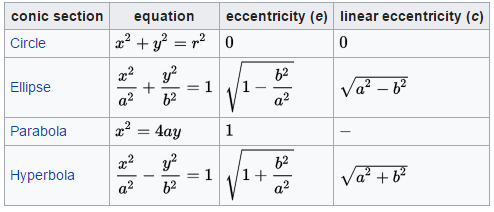# Newton’s Laws of Motion and Kepler’s Laws

Newton’s Laws:

1. An object at rest remains at rest, and an object in motion remains in motion at constant speed and in a straight line unless acted on by an unbalanced force.

2. The acceleration of an object depends on the mass of the object and the amount of force applied.

3(!). Whenever one object exerts a force on another object, the second object exerts an equal and opposite on the first.

Kepler’s Laws:

1. Planetary orbits are elliptical with the sun at a focus.

2. The radius vector from the sun to a planet sweeps equal areas in equal times.

3. The ratio of the square of the period of revolution and the cube of the ellipse semimajor axis is the same for all planets.

# Basic Information

Consider a large body (the primary) with a sattelite orbiting around it. A satellite orbits a primary because of the gravity the primary exerts on the satellite. While the satellite is generally much smaller, it does also exert gravity on the primary according to the third law of motion.

The point where the satellite is closest to the primary is called the periapsis and this is where the satellite has the most energy. The point in the orbit where the satellite is furthest from the primary is called the apoapsis, and this is where the energy of the satellite is the lowest. In an orbit around Earth, apogee and perigee are the highest and lowest points.

# Newton’s Law of Universal Gravitation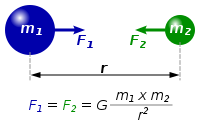Where:

• F is the force between the masses;
• G is the gravitational constant (6.674×10−11 m3⋅kg−1⋅s−2);
• m1 is the first mass;
• m2 is the second mass;
• r is the distance between the centers of the masses.

# Centripetal force

A centripetal force is a force that acts on a body moving in a circular path and is directed towards the centre around which the body is moving.Where:

•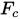is the centripetal force
• m is the mass
•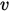is the velocity

# Circular Orbit

While perfectly circular orbits don’t really exist they can be really helpful in understanding on what is going on.

In this case, the satellite moves in a perfect circle around the primary at a distance r. Because it is a perfectly circular orbit, the force pulling the satellite towards the primary (gravity) is equal to the force trying to push the satellite away from it (centripetal force). By putting the functions for both these forces on either side of the equals sign, we can get the orbital velocity at a certain distance r: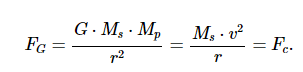Where :

• Ms is the mass of the sattelite
• Mp is the mass of the primary

We can cross out the mass of the sattelite and by multiplying both functions with r we have :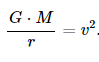Which can be written as :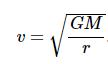Where G is 6.673 x 10-11 N•m2/kg2, Mp is the mass of the primary about which the satellite orbits, and r is the radius of orbit for the satellite.

The actual velocity can be also transformed into an angular velocity ω which gives us the following function: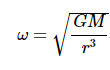# The Acceleration Equation

The acceleration value of a satellite is equal to the acceleration of gravity of the satellite at whatever location that it is orbiting. Thus the acceleration of a satellite in circular motion about some primary is given by the following equation: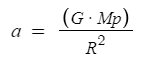# Third Law of Kepler

Let me remind you the function of the velocity of a sattelite moving in a circular orbit.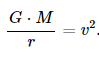Now, when an object is moving in a circle, we can get its speed by the following equation: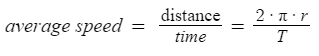Where:

• R represents the radius of the circle
• T represents Period

Let’s see what we have by substituting for υ :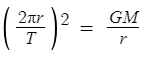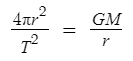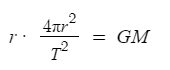Solving for T^2 we find: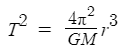By rearranging this equation we can find the constant of proportionality for Kepler’s Third law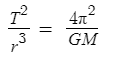The interesting thing of these equations is that none of them has as a variable the mass of the satellite in them. The period, speed and acceleration of a satellite are only dependent upon the radius of orbit and the mass of the central body that the satellite is orbiting.

# But what about other eccentricities?

As I have stated before, circular orbit does not exist in reality. But then what happens with other orbits, like the elliptical ones?

# Elliptic Orbit

An elliptic orbit or elliptical orbit is an orbit with an eccentricity of less than 1 and greater than 0.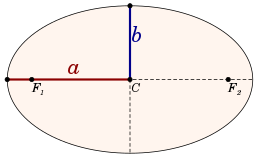Just as the circular orbits are described by a circle around its center, elliptical orbits are described by an ellipse around two foci. The major axis of an ellipse is its longest diameter, a line segment that runs through the center and both foci, with ends at the two most widely separated points of the perimeter. The semi-major axis is the line through the two foci. The semi-minor axis of an ellipse is the line through the center of the ellipse orthogonal to the semi-major axis. In the image the semi-major axis is (a) and the semi-minor axis is (b).

The distance from the center to one of the foci c is called the linear eccentricity. By dividing this with the distance a, you get the eccentricity of the ellipse, which is the ratio of the distance c between the center of the ellipse and each focus to the length of the semimajor axis a.

By adding the apoapsis and periapsis heights, you should get exactly 2a. If we also know the centre of the ellipse (it’s between apoapsis and periapsis), we can calculate the distance between the centre and the primary to obtain c. By having a and c we can calculateo calculate the eccentricity e.

# Orbital Elements

• eccentricity (e),
• semi-major axis (a),
• inclination (i),
• longitude of the ascending node (Ω),
• argument of periapsis (ω),
• true anomaly (ν)
• reference direction
• periapsis
• apoasis

# Lua Example for a circular earth orbit

alphabot22/Satellite-Calculations: Some simple satellite calculations in lua (github.com)

Main:

``````local G = script.Parent.Configuration.Constants.G
local Rcentral = script.Parent.Configuration.Constants.Rearth
local M = script.Parent.Configuration.Constants.M

function cubeRoot(x : number)
return (x ^ (1 / 3))
end

local Orbit = {}
Orbit._index = Orbit

function Orbit.findSatelliteVelocity(height:number)
local R = Rcentral + height
local velocity = math.sqrt(G*M/R)
return velocity
end

function Orbit.findSatelliteAcceleration(height:number)
local R = Rcentral + height
local v = Orbit.findSatelliteVelocity(height)
local a = v^2/R
return a
end

function Orbit.findSatellitePeriod(height : height)
local R = Rcentral + height
local T = math.sqrt(4* math.pi^2 * R^3 / G*M)
return T
end

function Orbit.findSatelliteHeight(period : number)
local T = period
local Rcube = T^2 * G * M / 4 * math.pi^2
local R = cubeRoot(Rcube)
local h = R - Rcentral
return h
end

return Orbit
``````

Constants:

``````local module = {

G = 6.67408 * 10^-20,

Rearth = 6.37*10^6 ,

M = 5.98*10^24
}

return module
``````
6 Likes